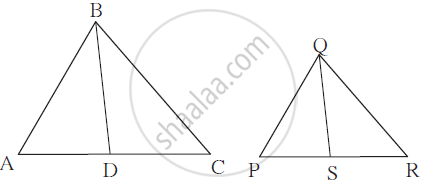Advertisement Remove all ads

# In δ Abc and δ Pqr, ∠ Abc ≅ ∠ Pqr, Seg Bd and Seg Qs Are Angle Bisector. I F L ( a D ) L ( P S ) = L ( D C ) L ( S R ) Prove that : δ Abc ∼ δ Pqr - Geometry

In Δ ABC and Δ PQR,
∠ ABC ≅ ∠ PQR, seg BD and
seg QS are angle bisector.
If  (l(AD))/(l(PS)) = (l(DC))/(l(SR))
Prove that : Δ ABC ∼ Δ PQRAdvertisement Remove all ads

#### Solution

Proof : (l(AD))/(l(PS)) = (l(DC))/(l(SR)) ∴ (l(AD))/(l(DC)) = (l(PS))/(l(SR))
According to angle bisector theorem, (l(AD))/(l(DC)) = (l(AB))/(l(BC)) ; (l(PS))/(l(SR)) = (l(PQ))/((QR))
∴ (l(AB))/(l(BC)) = (l(PQ))/(l(QR)) and ∠ ABC ≅ ∠ PQR ..... (Given)
Δ ABC ∼ Δ PQR ........ (SAS Test)

Concept: Property of an Angle Bisector of a Triangle
Is there an error in this question or solution?
Advertisement Remove all ads
Advertisement Remove all ads
Share
Notifications

View all notifications

Forgot password?